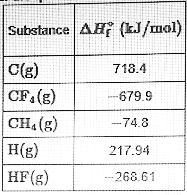# Problem: Part A. Calculate the enthalpy change, delta H, for the expansion of methaneCH4(g) → C(g) + 4H(g)Part B. Calculate the enthalpy change, delta H for the reverse of the formation of methane.CH4(g) → C(s) + 2H2(g)Part C. Suppose that 0.570 mol of methane CH 4(g) is reacted with 0.720 mol of fluorine, F2(g), forming CF4(g) and HF (g) as sole products. Assuming that the reaction occurs at constant pressure, how much heat is released?Use the data below to answer the questions.

###### FREE Expert Solution

PART A

We’re being asked to determine the standard enthalpy change (ΔH˚rxn) for the balanced reaction:

CH4(g) → C(g) + 4H(g)

Recall that ΔH˚rxn can be calculated from the enthalpy of formation (ΔH˚f) of the reactants and products involved:

92% (265 ratings)###### Problem Details

Part A. Calculate the enthalpy change, delta H, for the expansion of methane

CH4(g) → C(g) + 4H(g)

Part B. Calculate the enthalpy change, delta H for the reverse of the formation of methane.

CH4(g) → C(s) + 2H2(g)

Part C. Suppose that 0.570 mol of methane CH 4(g) is reacted with 0.720 mol of fluorine, F2(g), forming CF4(g) and HF (g) as sole products. Assuming that the reaction occurs at constant pressure, how much heat is released?

Use the data below to answer the questions.